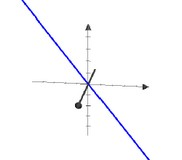# Trigonometry - Angle (or Arc) (Alpha - α)

Angle is counted in the Babylonian numeral system, base-60. (360 degrees in a circle)

## Illustration

with the identity function

• seconds

## Notation

````10°25'59" `
```

where:

Right angle = 90 degree

Discover More(Geometry|Linear Algebra) - Euclidean Space

In geometry, Euclidean space encompasses: the two-dimensional Euclidean plane, the three-dimensional space of Euclidean geometry, and certain other spaces. The term “Euclidean” distinguishes...(Turn|Cycle|Revolution|Full Circle|Complete Rotation)

A Turn is a Unit ofPlane angle. A turn is also known as: a cycle (abbreviated cyc), a revolution (abbreviated rev), a complete rotation (abbreviated rot) or full circle. 1 turn = 2π...Angle - Minute

One minute is a unit notation of angle where 1 degree = 60 minutes Minute_and_second_of_arcData Mining - Cosine Similarity (Measure of Angle)

The cosine similarity is a measure of the angle between two vectors, normalized by magnitude. You just divide the dot product by the magnitude of the two vectors. By taking the and definition of...Decimal - Fraction

A decimal fraction is a fraction in the decimal numeral system (base 10). They are also known as the decimals. In 999.99, the fraction or decimals are 99 Time and angles use decimal fractions of...Geometry - Object

in Geometry An object can be: a pre-defined shape or an arbitrary one defined with the help of a path. Notions such as: The length of a vector The angle between two vectors. are introduced...Geometry - Perpendicular (Right angle)

In elementary geometry, the word perpendicular describes the relationship between two geometric objects that meet at a right angle.Number - Babylonian numeral system, base-60

The sexagesimal numeral system or base-60, was the first positional system in the first language developed the Cuneiform It was used by astronomers Numeral System Parts Babylonian numerals and other...Trignometry - Identity function (sin2+cos2=1)

identify function in Trignometry where: Other illustration : The identity function is a derivative from the pythagorean...Trigonometry

(from Greek trigōnon, triangle and metron, “measure”) is a branch of mathematics that studies relationships involving: lengths and angles of triangles.